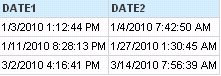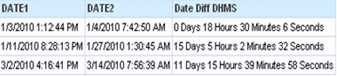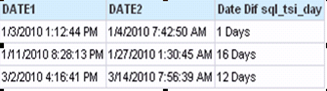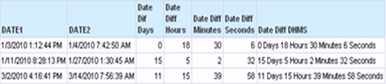# KPI Partners Blog

### by Kurt Wolff

Suppose you have a series of datetime pairs in Oracle BI... The problem is to display the differences between DATE1 and DATE2 in days, hours, minutes, and seconds.There are undoubtedly multiple solutions to this problem, and you might want to try solve it yourself before reading the approach in this post.  If you do, here's some Oracle SQL to create and populate a table with the base data.
CREATE TABLE TIMECALCTABLE
(    RNUM NUMBER,
DATE1 DATE,
DATE2 DATE
)
insert into TimeCalcTable (RNum, Date1, Date2) values (1, to_date('03-Jan-10 13:12:44','dd-Mon-yy hh24:mi:ss'), to_date('04-Jan-10 07:42:50', 'dd-Mon-yy hh24:mi:ss')); insert into TimeCalcTable(RNum, Date1, Date2) values (2, to_date('11-Jan-10 20:28:13','dd-Mon-yy hh24:mi:ss'),to_date('27-Jan-10 01:30:45', 'dd-Mon-yy hh24:mi:ss')); insert into TimeCalcTable(RNum, Date1, Date2) values (3, to_date('02-Mar-10 16:16:41','dd-Mon-yy hh24:mi:ss'),to_date('14-Mar-10 07:56:39', 'dd-Mon-yy hh24:mi:ss'));
If you tried this, you might have been tempted to start by first calculating the number of days between the two dates. If you used the timestampdiff function with the sql_tsi_day parameter, you would have seen results like this:The physical SQL generated for the calculation using the Oracle database is
Trunc(DATE2) - Trunc(DATE1)
– i.e. midnight of DATE2 minus midnight of DATE1.

So, in the first row, it calculates a 1 day difference between DATE1 and DATE2, even though there is less than a 24 hour period between them.

To use the Timestampdiff function to get accurate results, you need to calculate the difference in days using a smaller time parameter, e.g. sql_tsi_minute. The formula below uses the Timestampdiff function to return the number of minutes between the two columns, then divides that number by the number of minutes in a day (24 hours times 60 minutes) discarding any remainder with the floor function.
FLOOR( TIMESTAMPDIFF( SQL_TSI_MINUTE , "DATE1", "DATE2") / (24 * 60))

To calculate how many hours are left that are not included in days, take the difference in minutes between the two columns,  divide that by 60 to get hours, discard the remainder with the floor function, then subtract the number of hours already represented in days.

FLOOR( TIMESTAMPDIFF( SQL_TSI_MINUTE , "DATE1","DATE2") / 60) - 24 *
FLOOR( TIMESTAMPDIFF( SQL_TSI_MINUTE , "DATE1", "DATE2") / (24 * 60))

To calculate the number of minutes, find the difference in seconds, divide by 60, use the floor function to discard any remainder,  and subtract the minutes that have already been represented in hours and days.
FLOOR( TIMESTAMPDIFF( SQL_TSI_SECOND ,"DATE1","DATE2") / 60)
- 60 *  FLOOR( TIMESTAMPDIFF( SQL_TSI_MINUTE , "DATE1","DATE2") / 60)

Finally, to calculate seconds, calculate the difference in seconds and discard the seconds accounted for in minutes, hours, and days (i.e. the seconds in the whole minutes between the two columns).
TIMESTAMPDIFF( SQL_TSI_SECOND , "DATE1","DATE2") - 60 *  FLOOR( TIMESTAMPDIFF( SQL_TSI_SECOND , "DATE1", "DATE2") / 60)

Now you can combine these formulas, casting results as varchars, and add text to show the time durations of days, hours, minutes, and seconds.

cast(floor(TimestampDiff(SQL_TSI_MINUTE, DATE1, DATE2) / (24 * 60)) as  VARCHAR ( 8 ))  || ' Days' || ' ' || cast(floor(TimestampDiff(SQL_TSI_MINUTE, DATE1, DATE2) /  60) - floor(TimestampDiff(SQL_TSI_MINUTE, DATE1, DATE2) / (24 * 60) * 24 as  VARCHAR ( 8 ))  || ' Hours ' || cast(floor(TimestampDiff(SQL_TSI_SECOND, DATE1, DATE2) / 60) - floor(TimestampDiff(SQL_TSI_MINUTE, DATE1, DATE2) /  60) * 60 as  VARCHAR ( 8 ))  || ' Minutes ' || cast(TimestampDiff(SQL_TSI_SECOND, DATE1, DATE2) - floor(TimestampDiff(SQL_TSI_SECOND, DATE1, DATE2) / 60) * 60 as  VARCHAR ( 8 ))  || ' Seconds'Tags: Kurt Wolff, Oracle BI, Blog